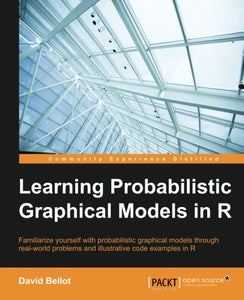# Learning Probabilistic Graphical Models In R

• Publish Date: 2016-04-29
• Binding: Paperback
• Author: David Bellot
Regular price \$52.88

Attention:For textbook, access codes and supplements are not guaranteed with used items.

#### Key Features

• Predict and use a probabilistic graphical models (PGM) as an expert system
• Comprehend how your computer can learn Bayesian modeling to solve real-world problems
• Know how to prepare data and feed the models by using the appropriate algorithms from the appropriate R package

#### Book Description

Probabilistic graphical models (PGM, also known as graphical models) are a marriage between probability theory and graph theory. Generally, PGMs use a graph-based representation. Two branches of graphical representations of distributions are commonly used, namely Bayesian networks and Markov networks. R has many packages to implement graphical models.

We'll start by showing you how to transform a classical statistical model into a modern PGM and then look at how to do exact inference in graphical models. Proceeding, we'll introduce you to many modern R packages that will help you to perform inference on the models. We will then run a Bayesian linear regression and you'll see the advantage of going probabilistic when you want to do prediction.

Next, you'll master using R packages and implementing its techniques. Finally, you'll be presented with machine learning applications that have a direct impact in many fields. Here, we'll cover clustering and the discovery of hidden information in big data, as well as two important methods, PCA and ICA, to reduce the size of big problems.

#### What you will learn

• Understand the concepts of PGM and which type of PGM to use for which problem
• Tune the model's parameters and explore new models automatically
• Understand the basic principles of Bayesian models, from simple to advanced
• Transform the old linear regression model into a powerful probabilistic model
• Use standard industry models but with the power of PGM
• Understand the advanced models used throughout today's industry
• See how to compute posterior distribution with exact and approximate inference algorithms

David Bellot is a PhD graduate in computer science from INRIA, France, with a focus on Bayesian machine learning. He was a postdoctoral fellow at the University of California, Berkeley, and worked for companies such as Intel, Orange, and Barclays Bank. He currently works in the financial industry, where he develops financial market prediction algorithms using machine learning. He is also a contributor to open source projects such as the Boost C++ library.